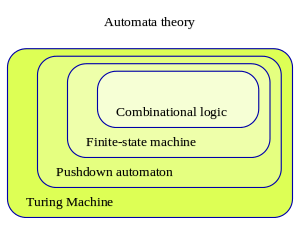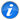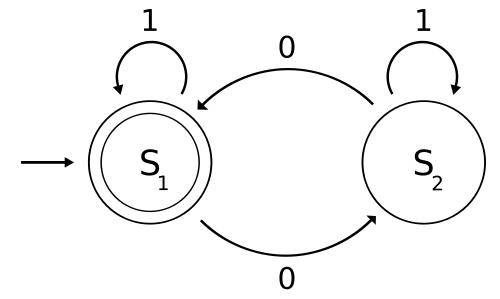# Automata theoryClasses of automataThe study of the mathematical properties of such automata is automata theory. The picture is a visualization of an automaton that recognizes strings containing an even number of 0s. The automaton starts in state S1, and transitions to the non-accepting state S2 upon reading the symbol 0. Reading another 0 causes the automaton to transition back to the accepting state S1. In both states the symbol 1 is ignored by making a transition to the current state.

Automata theory is the study of abstract machines and automata, as well as the computational problems that can be solved using them. It is a theory in theoretical computer science and discrete mathematics (a subject of study in both mathematics and computer science). The word automata (the plural of automaton) comes from the Greek word αὐτόματα, which means "self-acting".

The figure at right illustrates a finite-state machine, which belongs to a well-known type of automaton. This automaton consists of states (represented in the figure by circles) and transitions (represented by arrows). As the automaton sees a symbol of input, it makes a transition (or jump) to another state, according to its transition function, which takes the current state and the recent symbol as its inputs.

Automata theory is closely related to formal language theory. An automaton is a finite representation of a formal language that may be an infinite set. Automata are often classified by the class of formal languages they can recognize, typically illustrated by the Chomsky hierarchy which describes the relations between various languages and kinds of formalized logic.

Automata play a major role in theory of computation, compiler construction, artificial intelligence, parsing and formal verification.

## Automata

Following is an introductory definition of one type of automaton, which attempts to help one grasp the essential concepts involved in automata theory/theories.

### Informal description

An automaton is supposed to run on some given sequence of inputs in discrete time steps. An automaton gets one input every time step that is picked up from a set of symbols or letters, which is called an alphabet. At any time, the symbols so far fed to the automaton as input, form a finite sequence of symbols, which finite sequences are called words. An automaton contains a finite set of states. At each instance in time of some run, the automaton is in one of its states. At each time step when the automaton reads a symbol, it jumps or transitions to another state that is decided by a function that takes the current state and symbol as parameters. This function is called the transition function. The automaton reads the symbols of the input word one after another and transitions from state to state according to the transition function, until the word is read completely. Once the input word has been read, the automaton is said to have stopped and the state at which automaton has stopped is called the final state. Depending on the final state, it's said that the automaton either accepts or rejects an input word. There is a subset of states of the automaton, which is defined as the set of accepting states. If the final state is an accepting state, then the automaton accepts the word. Otherwise, the word is rejected. The set of all the words accepted by an automaton is called the language recognized by the automaton.

In short, an automaton is a mathematical object that takes a word as input and decides either to accept it or reject it. Since all computational problems are reducible into the accept/reject question on words (all problem instances can be represented in a finite length of symbols), automata theory plays a crucial role in computational theory.

### Formal definition

Automaton
A deterministic finite automaton is represented formally by a 5-tuple (Q,Σ,δ,q0,F), where:
• Q is a finite set of states.
• Σ is a finite set of symbols, called the alphabet of the automaton.
• δ is the transition function, that is, δ: Q × Σ  Q.
• q0 is the start state, that is, the state of the automaton before any input has been processed, where q0∈ Q.
• F is a set of states of Q (i.e. F⊆Q) called accept states.
Input word
An automaton reads a finite string of symbols a1,a2,...., an , where ai  Σ, which is called an input word. The set of all words is denoted by Σ*.
Run
A sequence of states q0,q1,q2,...., qn, where qi  Q such that q0 is the start state and qi = δ(qi-1,ai) for 0 < i  n, is a run of the automaton on an input word w = a1,a2,...., an  Σ*. In other words, at first the automaton is at the start state q0, and then the automaton reads symbols of the input word in sequence. When the automaton reads symbol ai it jumps to state qi = δ(qi-1,ai). qn is said to be the final state of the run.
Accepting word
A word w  Σ* is accepted by the automaton if qn  F.
Recognized language
An automaton can recognize a formal language. The language L  Σ* recognized by an automaton is the set of all the words that are accepted by the automaton.
Recognizable languages
The recognizable languages are the set of languages that are recognized by some automaton. For the above definition of automata the recognizable languages are regular languages. For different definitions of automata, the recognizable languages are different.

## Variant definitions of automata

Automata are defined to study useful machines under mathematical formalism. So, the definition of an automaton is open to variations according to the "real world machine", which we want to model using the automaton. People have studied many variations of automata. The most standard variant, which is described above, is called a deterministic finite automaton. The following are some popular variations in the definition of different components of automata.

Input
• Finite input: An automaton that accepts only finite sequence of symbols. The above introductory definition only encompasses finite words.
• Infinite input: An automaton that accepts infinite words (ω-words). Such automata are called ω-automata.
• Tree word input: The input may be a tree of symbols instead of sequence of symbols. In this case after reading each symbol, the automaton reads all the successor symbols in the input tree. It is said that the automaton makes one copy of itself for each successor and each such copy starts running on one of the successor symbol from the state according to the transition relation of the automaton. Such an automaton is called a tree automaton.
• Infinite tree input : The two extensions above can be combined, so the automaton reads a tree structure with (in)finite branches. Such an automaton is called an infinite tree automaton
States
• Finite states: An automaton that contains only a finite number of states. The above introductory definition describes automata with finite numbers of states.
• Infinite states: An automaton that may not have a finite number of states, or even a countable number of states. For example, the quantum finite automaton or topological automaton has uncountable infinity of states.
• Stack memory: An automaton may also contain some extra memory in the form of a stack in which symbols can be pushed and popped. This kind of automaton is called a pushdown automaton
Transition function
• Deterministic: For a given current state and an input symbol, if an automaton can only jump to one and only one state then it is a deterministic automaton.
• Nondeterministic: An automaton that, after reading an input symbol, may jump into any of a number of states, as licensed by its transition relation. Notice that the term transition function is replaced by transition relation: The automaton non-deterministically decides to jump into one of the allowed choices. Such automata are called nondeterministic automata.
• Alternation: This idea is quite similar to tree automaton, but orthogonal. The automaton may run its multiple copies on the same next read symbol. Such automata are called alternating automata. Acceptance condition must satisfy all runs of such copies to accept the input.
Acceptance condition
• Acceptance of finite words: Same as described in the informal definition above.
• Acceptance of infinite words: an omega automaton cannot have final states, as infinite words never terminate. Rather, acceptance of the word is decided by looking at the infinite sequence of visited states during the run.
• Probabilistic acceptance: An automaton need not strictly accept or reject an input. It may accept the input with some probability between zero and one. For example, quantum finite automaton, geometric automaton and metric automaton have probabilistic acceptance.

Different combinations of the above variations produce many classes of automaton.

Automata theory is a subject matter that studies properties of various types of automata. For example, the following questions are studied about a given type of automata.

• Which class of formal languages is recognizable by some type of automata? (Recognizable languages)
• Are certain automata closed under union, intersection, or complementation of formal languages? (Closure properties)
• How much is a type of automata expressive in terms of recognizing a class of formal languages? And, their relative expressive power? (Language Hierarchy)

Automata theory also studies if there exist any effective algorithm or not to solve problems similar to the following list.

• Does an automaton accept any input word? (emptiness checking)
• Is it possible to transform a given non-deterministic automaton into deterministic automaton without changing the recognizable language? (Determinization)
• For a given formal language, what is the smallest automaton that recognizes it? (Minimization).

## Classes of automata

The following is an incomplete list of types of automata.

Automaton Recognizable language
Nondeterministic/Deterministic Finite state machine (FSM) regular languages
Deterministic pushdown automaton (DPDA) deterministic context-free languages
Pushdown automaton (PDA) context-free languages
Linear bounded automaton (LBA) context-sensitive languages
Turing machine recursively enumerable languages
Deterministic Büchi automaton ω-limit languages
Nondeterministic Büchi automaton ω-regular languages
Rabin automaton, Streett automaton, Parity automaton, Muller automaton ω-regular languages

### Discrete, continuous, and hybrid automata

Normally automata theory describes the states of abstract machines but there are analog automata or continuous automata or hybrid discrete-continuous automata, which use analog data, continuous time, or both.

## Hierarchy in terms of powers

The following is an incomplete hierarchy in terms of powers of different types of virtual machines.

Automaton
Deterministic Finite Automaton (DFA) -- Lowest Power

(same power)(same power)
Nondeterministic Finite Automaton (NFA)
(above is weaker)(below is stronger)
Deterministic Push Down Automaton (DPDA-I)
with 1 push-down storeNondeterministic Push Down Automaton (NPDA-I)
with 1 push-down storeLinear Bounded Automaton (LBA)Deterministic Push Down Automaton (DPDA-II)
with 2 push-down storesNondeterministic Push Down Automaton (NPDA-II)
with 2 push-down storesDeterministic Turing Machine (DTM)Nondeterministic Turing Machine (NTM)Probabilistic Turing Machine (PTM)Multitape Turing Machine (MTM)Multidimensional Turing Machine—Highest Power

## Applications

Each model in AT plays important roles in several applied areas. Finite automata are used in text processing, compilers, and hardware design. Context-free grammar (CFGs) are used in programming languages and artificial intelligence. Originally, CFGs were used in the study of the human languages. Cellular automata are used in the field of biology, the most common example being John Conway's Game of Life. Some other examples which could be explained using automata theory in biology include mollusk and pine cones growth and pigmentation patterns. Going further, a theory suggesting that the whole universe is computed by some sort of a discrete automaton, is advocated by some scientists. The idea originated in the work of Konrad Zuse, and was popularized in America by Edward Fredkin.

## Automata simulators

Automata simulators are pedagogical tools used to teach, learn and research automata theory. An automata simulator takes as input the description of an automaton and then simulates its working for an arbitrary input string. The description of the automaton can be entered in several ways. An automaton can be defined in a symbolic language or its specification may be entered in a predesigned form or its transition diagram may be drawn by clicking and dragging the mouse. Well known automata simulators include Turing’s World, JFLAP, VAS, TAGS and SimStudio.

## Connection to category theory

One can define several distinct categories of automata following the automata classification into different types described in the previous section. The mathematical category of deterministic automata, sequential machines or sequential automata, and Turing machines with automata homomorphisms defining the arrows between automata is a Cartesian closed category, it has both categorical limits and colimits. An automata homomorphism maps a quintuple of an automaton Ai onto the quintuple of another automaton Aj. Automata homomorphisms can also be considered as automata transformations or as semigroup homomorphisms, when the state space,S, of the automaton is defined as a semigroup Sg. Monoids are also considered as a suitable setting for automata in monoidal categories.

Categories of variable automata

One could also define a variable automaton, in the sense of Norbert Wiener in his book on The Human Use of Human Beings via the endomorphisms. Then, one can show that such variable automata homomorphisms form a mathematical group. In the case of non-deterministic, or other complex kinds of automata, the latter set of endomorphisms may become, however, a variable automaton groupoid. Therefore, in the most general case, categories of variable automata of any kind are categories of groupoids or groupoid categories. Moreover, the category of reversible automata is then a 2-category, and also a subcategory of the 2-category of groupoids, or the groupoid category.

## References

1. Yan, Song Y. (1998). An Introduction to Formal Languages and Machine Computation. Singapore: World Scientific Publishing Co. Pte. Ltd. pp. 155–156.
2. Chakraborty, P., Saxena, P. C., Katti, C. P. 2011. Fifty Years of Automata Simulation: A Review. ACM Inroads, 2(4):59–70. http://dl.acm.org/citation.cfm?id=2038893&dl=ACM&coll=DL&CFID=65021406&CFTOKEN=86634854
3. Jirí Adámek and Vera Trnková. 1990. Automata and Algebras in Categories. Kluwer Academic Publishers:Dordrecht and Prague
4. S. Mac Lane, Categories for the Working Mathematician, Springer, New York (1971)
5. Cartesian closed category Archived November 16, 2011, at the Wayback Machine.
6. The Category of Automata Archived September 15, 2011, at the Wayback Machine.
7. http://www.csee.wvu.edu/~jworthing/asl2010.pdf James Worthington.2010.Determinizing, Forgetting, and Automata in Monoidal Categories. ASL North American Annual Meeting,March 17, 2010
8. Aguiar, M. and Mahajan, S.2010. "Monoidal Functors, Species, and Hopf Algebras".
9. Meseguer, J., Montanari, U.: 1990 Petri nets are monoids. Information and Computation 88:105–155